一不小心就弄懂了 冒泡，选择，插入，希尔,归并和快速排序

1 篇文章 1 订阅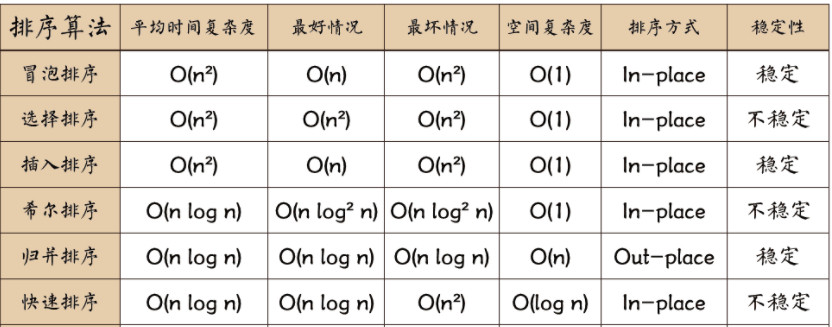🎈 常数阶Ο(1)
🎈 对数阶Ο(log2n)
🎈 线性阶Ο(n)
🎈 线性对数阶Ο(nlog2n)
🎈 平方阶Ο(n²)
🎈 立方阶Ο(n³)
🎈 K次方阶Ο(n^k)
🎈 指数阶Ο(2^n)Ο(1)＜Ο(log2n)＜Ο(n)＜Ο(nlog2n)＜Ο(n²)＜Ο(n³)＜…＜Ο(2^n)
＜Ο(n!)<O(n^n)

💓 冒泡排序（Quicksort）

①. 比较相邻的元素。如果第一个比第二个大，就交换他们两个。
②. 对每一对相邻元素作同样的工作，从开始第一对到结尾的最后一对。这步做完后，最后的元素会是最大的数。
③. 针对所有的元素重复以上的步骤，除了最后一个。
④. 持续每次对越来越少的元素重复上面的步骤①~③，直到没有任何一对数字需要比较。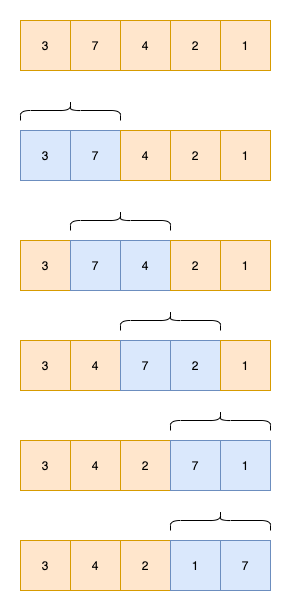public static void bubbleSort(int[] arr) {
// 进行i轮比较
for (int i = 0; i < arr.length - 1; i++)
{
for (int j = 0; j < arr.length - 1 - i; j++) {
//后一位的值大于前一位的值进行值交换
if (arr[j] > arr[j + 1]) {
swap(arr, j, j + 1);
}
}
}
}

public static void swap(int[] arr, int i, int j) {
int temp = arr[i];
arr[i] = arr[j];
arr[j] = temp;
}

🧡 选择排序（Quicksort）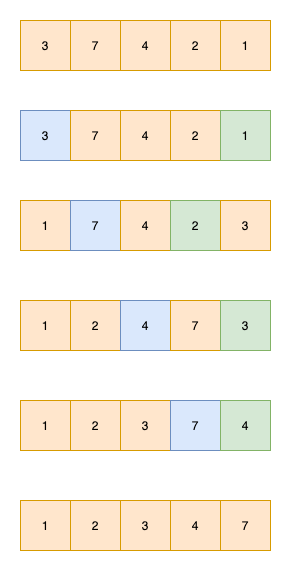public static void selectionSort(int[] a) {
//每当完成一轮，将会找到最小值，一个i代表一轮
for (int i = 0; i < a.length; i++) {
int index = i;
//每一轮从i+1开始找，查找是否有比当前值更小的值
for (int j = i + 1; j < a.length; j++) {
if (a[index] > a[j]) {
index = j;
}
}//如果index和i不相等说明，下标交换过，也就是说找到更小的数值了
if (index != i) {
swap(a, index, i);
}
}
}

public static void swap(int[] a, int i, int j) {
int temp = a[i];
a[i] = a[j];
a[j] = temp;
}

💛 插入排序（insertSort）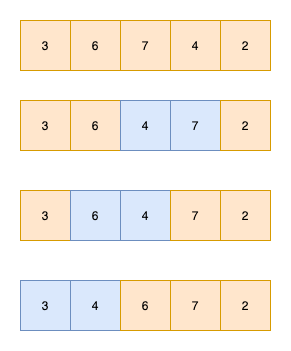public static void insertionSort(int[] a) {
for (int i = 1; i < a.length; i++) {
int temp = a[i];
int j;
// 查到合适的插入位置，插入即可
for (j = i - 1; j >= 0 && a[j] > temp; j--) {
a[j + 1] = a[j];
}
a[j + 1] = temp;
}
}

💚 希尔排序 （ShellSort）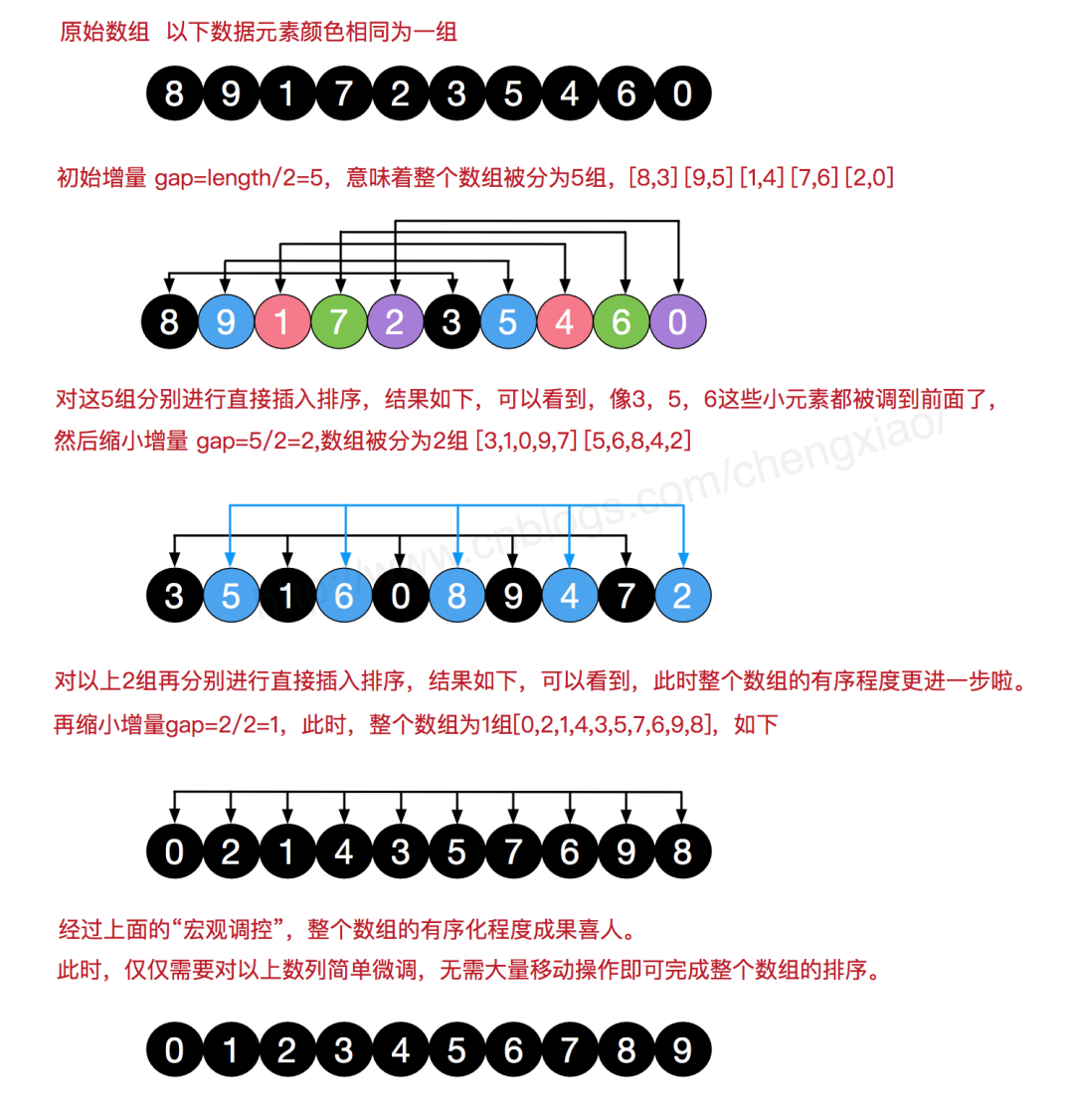public static void shellSort(int[] a) {
for (int step = a.length / 2; step > 0; step /= 2) {
//接下来的过程类似于插入排序
for (int i = step; i < a.length; i++) {
int temp = a[i];
int j;
for (j = i - step; j >= 0 && a[j] > temp ; j -= step) {
a[j + step] = a[j];
}
a[j + step] = temp;
}
}
}

💙 归并排序（MergetSort）

1.申请空间，使其大小为两个已经排序序列之和，该空间用来存放合并后的序列；

2.设定两个指针，最初位置分别为两个已经排序序列的起始位置；

3.比较两个指针所指向的元素，选择相对小的元素放入到合并空间，并移动指针到下一位置；

4.重复步骤 3 直到某一指针达到序列尾；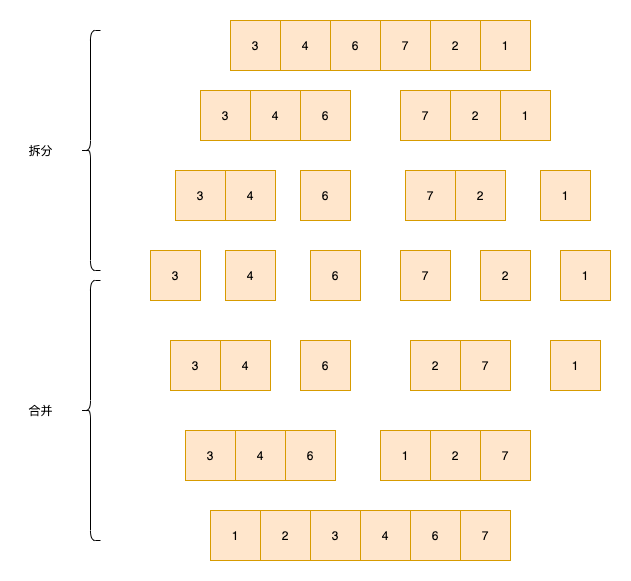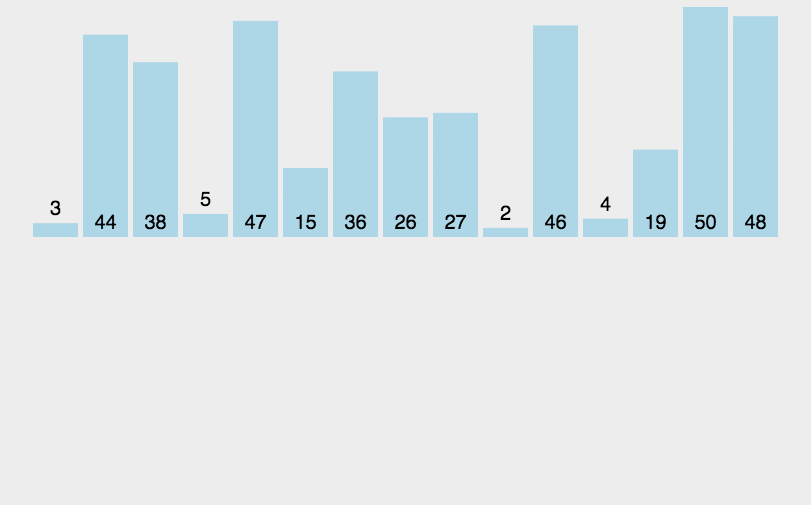public static void mergeSort(int[] a, int left, int right) {
// 将数组分段成只有一个元素
if (left == right) {
return;
}
int mid = (left + right) / 2;
mergeSort(a, left, mid);//递归
mergeSort(a, mid + 1, right);
merge(a, left, mid, right);
}

public static void merge(int[] a, int left, int mid, int right) {
int[] temp = new int[right - left + 1];
int i = left;
int j = mid + 1;
int k = 0;
while (i <= mid && j <= right) {
if (a[i] < a[j]) {
temp[k++] = a[i++];
} else {
temp[k++] = a[j++];
}
}
// 复制左边数组剩余的值
while (i <= mid) {
temp[k++] = a[i++];
}
// 复制右边数组剩余的值
while (j <= right) {
temp[k++] = a[j++];
}
int index = 0;
//把temp全部复制给数组
while (left <= right) {
a[left++] = temp[index++];
}
}

💜 快速排序（QuickSort）

1. 快速排序的执行流程主要分为如下三步
2. 从数列中取出一个数作为基准数
3. 分区，将比它大的数全放到它的右边，小于或等于它的数全放到它的左边
4. 再对左右区间重复第二步，直到各区间只有一个数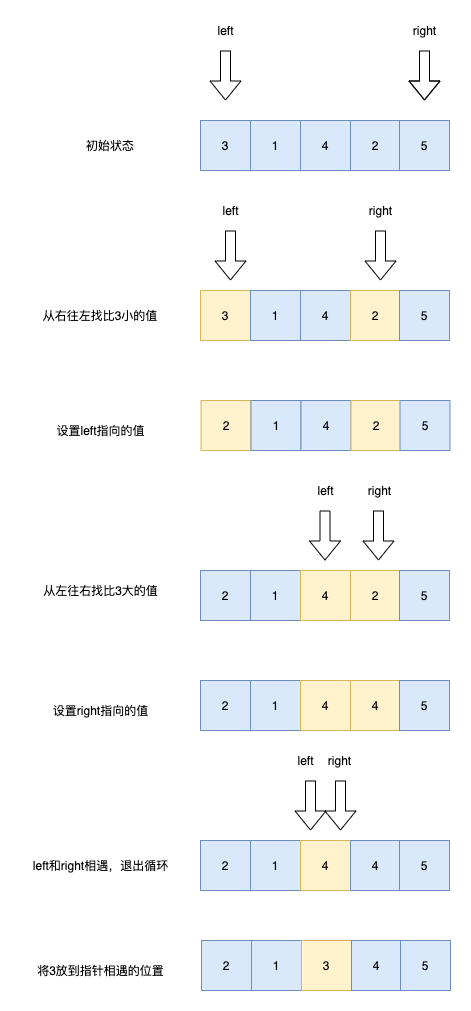public static void quickSort(int[] a, int left, int right) {
if (left >= right) {
return;
}
int index = sort(a, left, right);
//得到中间值index，然后一分为二，继续分
quickSort(a, left, index - 1);
quickSort(a, index + 1, right);
}

public static int sort(int[] a, int left, int right) {
//以左边的a[left]为基准数
int key = a[left];
while (left < right) {
// 从right所指位置向前搜索找到第一个关键字小于key的记录和key互相交换
while (left < right && a[right] >= key) {
right--;
}
a[left] = a[right];
// 从left所指位置向后搜索，找到第一个关键字大于key的记录和key互相交换
while (left < right && a[left] <= key) {
left++;
}
a[right] = a[left];
}
// 放key值，此时left和right相同
a[left] = key;
return left;
}
08-11286
08-09811

11-08130
07-1212
08-15318
05-20111
07-091138
12-14305
12-2442
11-04457
04-301420
05-08157
03-11151
09-06128
09-21236
01-13483
11-06156¥2 ¥4 ¥6 ¥10 ¥20余额支付 (余额：-- )扫码支付获取中扫码支付点击重新获取扫码支付1.余额是钱包充值的虚拟货币，按照1:1的比例进行支付金额的抵扣。
2.余额无法直接购买下载，可以购买VIP、C币套餐、付费专栏及课程。余额充值